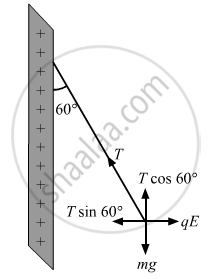Department of Pre-University Education, KarnatakaPUC Karnataka Science Class 12
Share

# Consider the Situation of the Previous Problem. (A) Find the Tension in the String in Equilibrium. - Physics

ConceptElectric Field Introduction of Electric Field

#### Question

One end of a 10 cm long silk thread is fixed to a large vertical surface of a charged non-conducting plate and the other end is fastened to a small ball of mass 10 g and a charge of 4.0× 10-6 C. In equilibrium, the thread makes an angle of 60° with the vertical (a) Find the tension in the string in equilibrium. (b) Suppose the ball is slightly pushed aside and released. Find the time period of the small oscillations.

#### Solution(a)
In equilibrium state, the thread makes an angle of 60o with the vertical.
The tension in the thread is resolved into horizontal and vertical components.
Then, tension in the string in equilibrium,

T cos 60° = mg

"T" xx 1/2 = (10 xx 10^-3) xx 10

T =(10 × 10-3 )× 10 × 2 = 0.20 N

(b) As it is displaced from equilibrium, net force on the ball,

"F" = sqrt (("mg")^2 + ( ("q" sigma)/(2∈_0))^2)

As F = ma

"a" = sqrt (("g"))^2 + (("q" sigma)/("m"2∈_0))^2

The surface charge density of the plate (as calculated in the previous question), σ = 7.5×10-7 C/m2
Charge on the ball, q = 4×10-6 C
Mass of the ball, m =
The time period of oscillation of the given simple pendulum,

"T" = 2 pi sqrt ("l"/"g")

= 2 pi sqrt ((10 xx 10^-2)/9.8)

= 0.45 sec

Is there an error in this question or solution?

#### APPEARS IN

Solution Consider the Situation of the Previous Problem. (A) Find the Tension in the String in Equilibrium. Concept: Electric Field - Introduction of Electric Field.
S